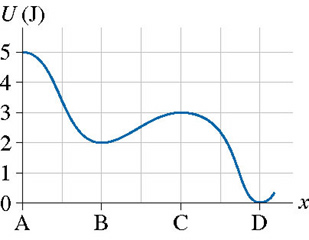# Problem: The figure is the potential-energy diagram for a 500 g particle that is released from rest at A. What are the particle's speeds at B, C, and D?

###### FREE Expert Solution

From the equation of kinetic energy:

K.E = (1/2)mv2

At point B:

The potential energy is 2J. The kinetic energy is (5 - 2) = 2 J.

81% (283 ratings)###### Problem Details

The figure is the potential-energy diagram for a 500 g particle that is released from rest at A. What are the particle's speeds at B, C, and D?Frequently Asked Questions

What scientific concept do you need to know in order to solve this problem?

Our tutors have indicated that to solve this problem you will need to apply the Force & Potential Energy concept. You can view video lessons to learn Force & Potential Energy. Or if you need more Force & Potential Energy practice, you can also practice Force & Potential Energy practice problems.

What professor is this problem relevant for?

Based on our data, we think this problem is relevant for Professor Li's class at UW-SEATTLE.